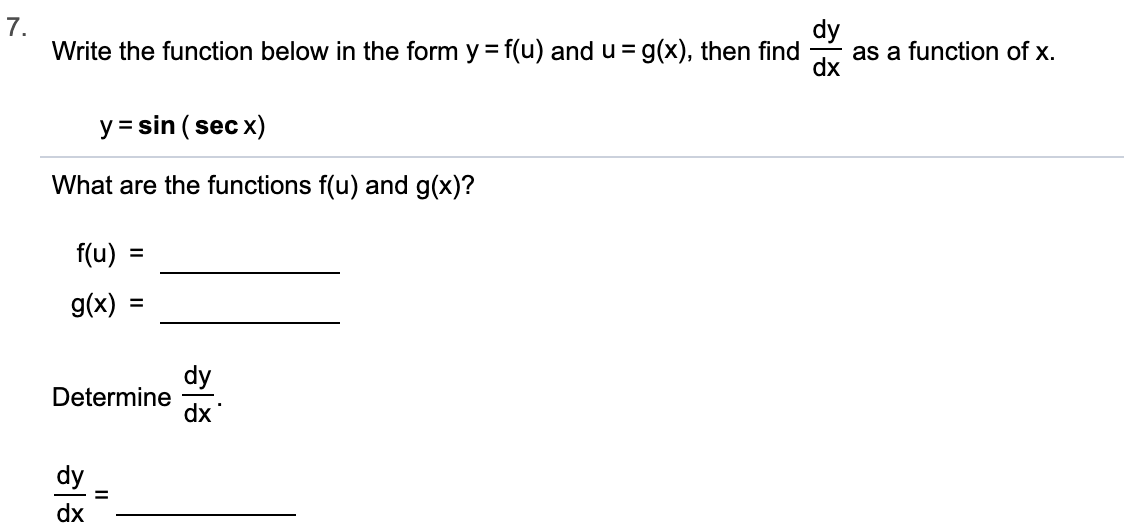# 7.Write the function below in the form y f(u) and u = g(x), then finddyas a function of x.dxysin (sec x)What are the functions f(u) and g(x)?f(u)g(x)dyDeterminedxdy1dx

Question
7 views

Can you help me step by step?help_outlineImage Transcriptionclose7. Write the function below in the form y f(u) and u = g(x), then find dy as a function of x. dx y sin (sec x) What are the functions f(u) and g(x)? f(u) g(x) dy Determine dx dy 1 dx fullscreen
check_circle

Step 1

y=f(u) and u=g(x) given

So y=f(g(x))

This means f will be outer function and g is the inner function

g(x)= sec x

Repla...

### Want to see the full answer?

See Solution

#### Want to see this answer and more?

Solutions are written by subject experts who are available 24/7. Questions are typically answered within 1 hour.*

See Solution
*Response times may vary by subject and question.
Tagged in

### Other